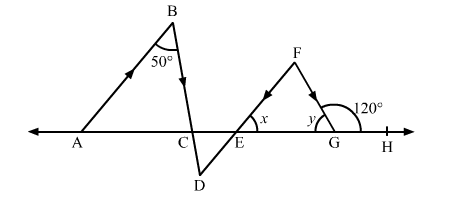# In the given figure, AB || DE and BD || FG such that ∠ABC = 50° and ∠FGH = 120°. Find the values of x and y.

Question:

In the given figure, AB || DE and BD || FG such that ∠ABC = 50° and ∠FGH = 120°. Find the values of x and y.Solution:

We have, ∠FGE + ∠FGH = 180°       (Linear pair of angles)

∴ y + 120° = 180°

⇒ = 180° − 120° = 60°

Now, AB || DF and BD is the transversal.

∴ ∠ABD = ∠BDF           (Pair of alternate angles)

⇒ ∠BDF = 50°

Also, BD || FG and DF is the transversal.

∴ ∠BDF = ∠DFG           (Pair of alternate angles)

⇒ ∠DFG = 50°       .....(1)

In ∆EFG,

∠FGH = ∠EFG + ∠FEG       (Exterior angle of a triangle is equal to the sum of the two opposite interior angles)

⇒ 120° = 50° + x                   [Using (1)]

⇒ x = 120° − 50° = 70°

Thus, the values of x and y are 70° and 60°, respectively.